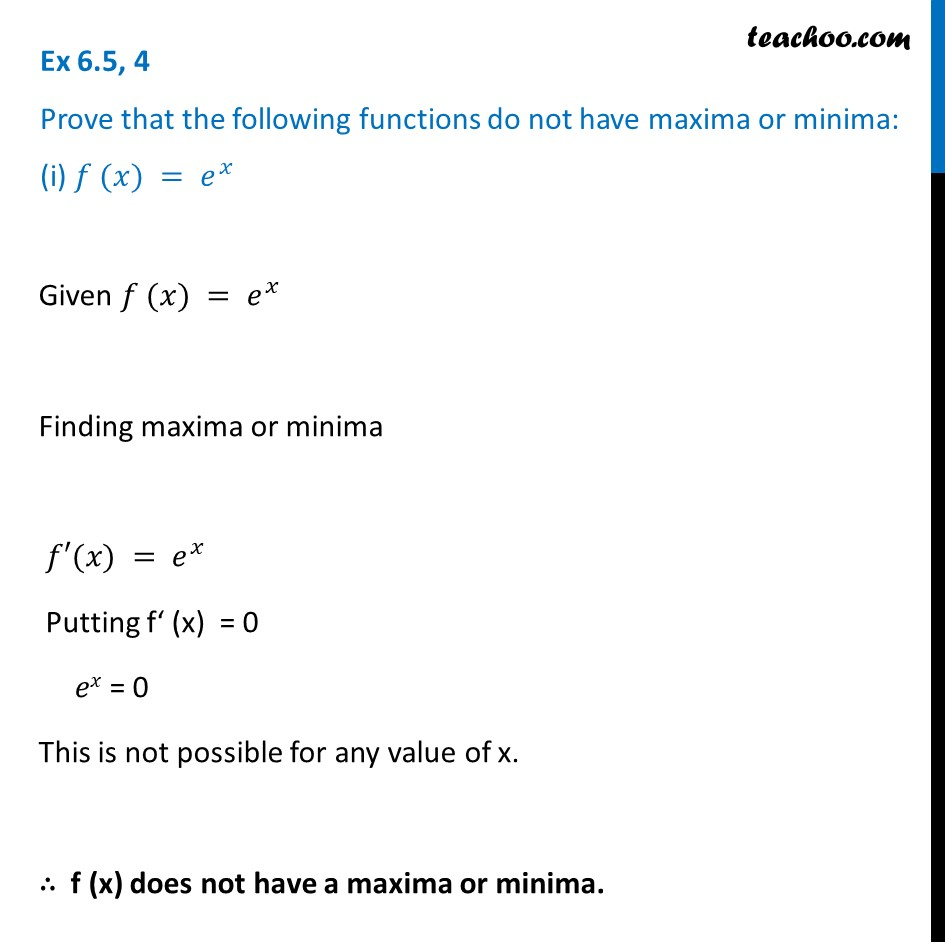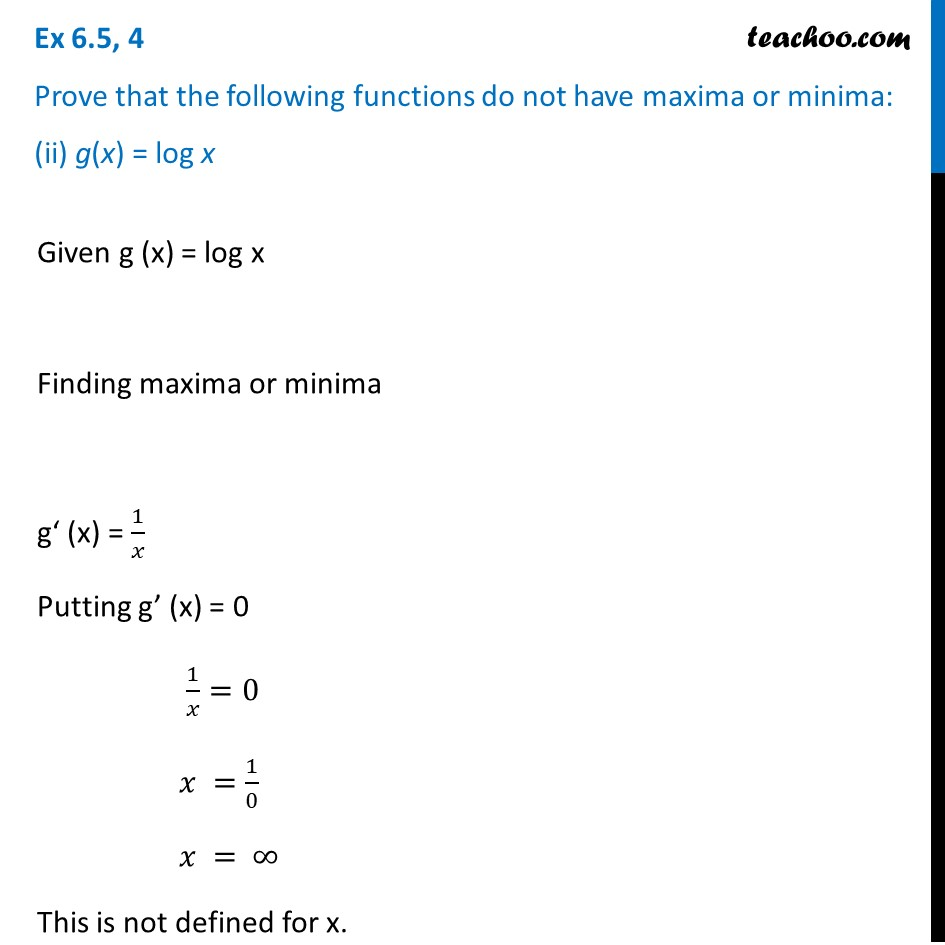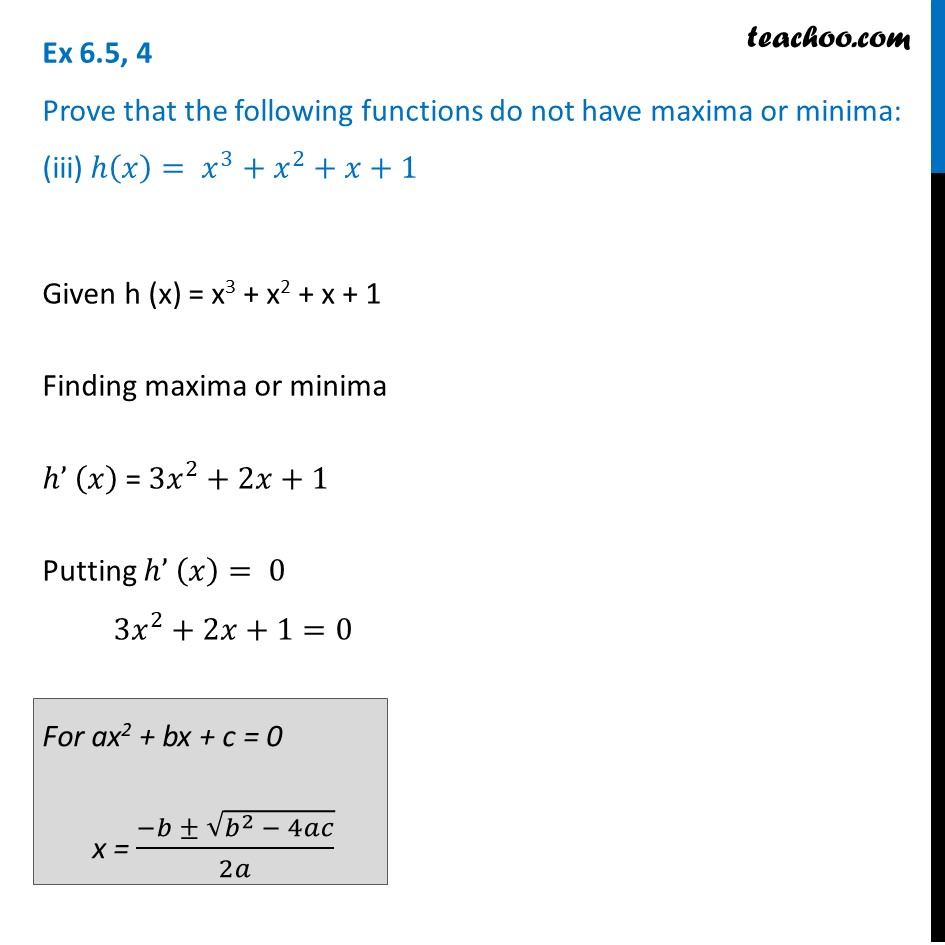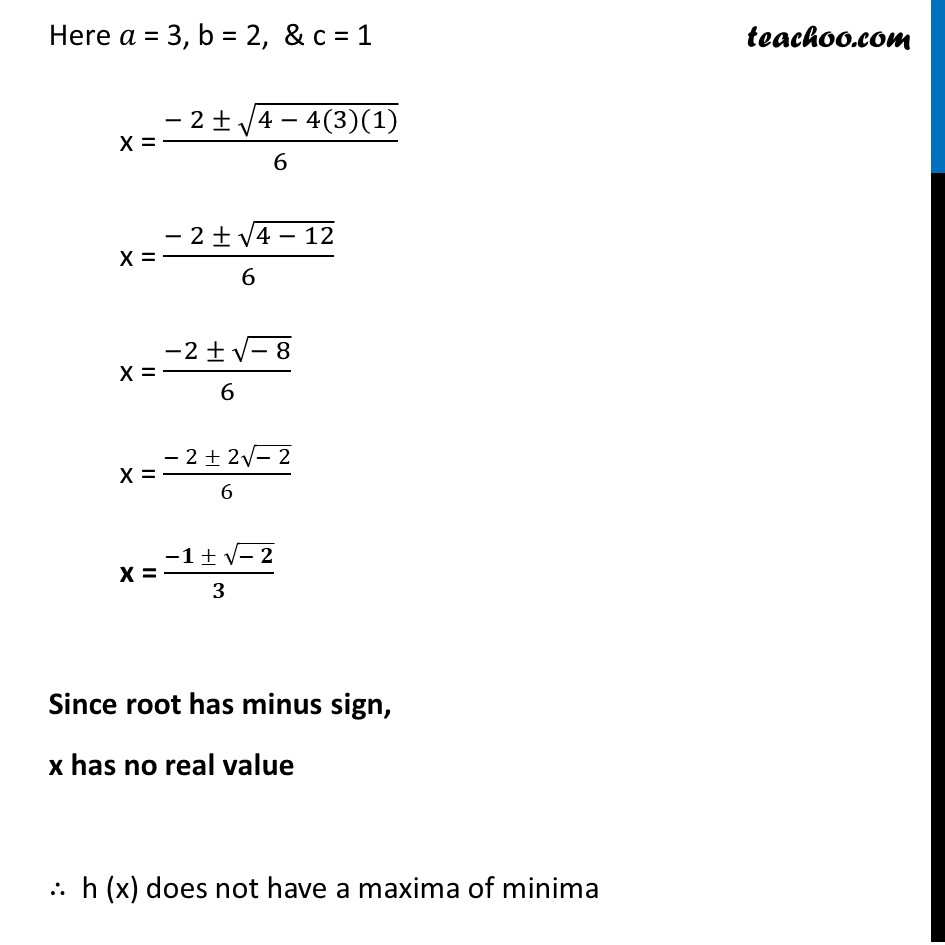1. Chapter 6 Class 12 Application of Derivatives (Term 1)
2. Serial order wise
3. Ex 6.5

Transcript

Ex 6.5, 4 Prove that the following functions do not have maxima or minima: (i) 𝑓 (𝑥) = 𝑒^𝑥Given 𝑓 (𝑥) = 𝑒^𝑥 Finding maxima or minima 𝑓′(𝑥) = 𝑒^𝑥 Putting f‘ (x) = 0 𝑒𝑥 = 0 This is not possible for any value of x. ∴ f (x) does not have a maxima or minima. Ex 6.5, 4 Prove that the following functions do not have maxima or minima: (ii) g(x) = log xGiven g (x) = log x Finding maxima or minima g‘ (x) = 1/𝑥 Putting g’ (x) = 0 1/𝑥=0 𝑥 =1/0 𝑥 = ∞ This is not defined for x. So, g (x) does not have a maxima or minima. Ex 6.5, 4 Prove that the following functions do not have maxima or minima: (iii) ℎ(𝑥)= 𝑥^3+𝑥^2+𝑥+1Given h (x) = x3 + x2 + x + 1 Finding maxima or minima ℎ’ (𝑥) = 3𝑥^2+2𝑥+1 Putting ℎ’ (𝑥)= 0 3𝑥^2+2𝑥+1=0 For ax2 + bx + c = 0 x = (−𝑏 ± √(𝑏^2 − 4𝑎𝑐))/2𝑎 Here 𝑎 = 3, b = 2, & c = 1 x = (− 2 ± √(4 − 4(3)(1)))/6 x = (− 2 ± √(4 − 12))/6 x = (−2 ± √(− 8))/6 x = (− 2 ± 2√(− 2))/6 x = (−𝟏 ± √(− 𝟐))/𝟑 Since root has minus sign, x has no real value ∴ h (x) does not have a maxima of minima

Ex 6.5